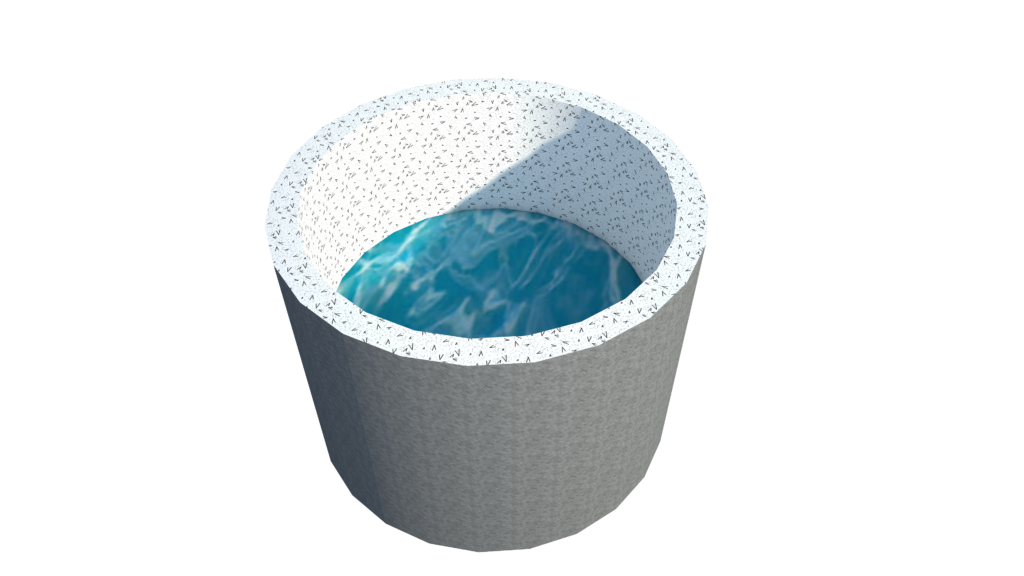# How To Calculate Circular Water Tank Size & Capacity

CIRCULAR WATER TANK SIZE CALCULATION FOR 2000 LITTERS WATERWater tank volume Capacity  = 2000 litters

Volume                                        V   = 2 meter Cube

Volume of water tank              V   = π r2 h

Assume water tank radius      r  = 0.5 m

Determine the size of circular water tank For 2000 liter water

V  = π r2 h

2  = π (0.5)2 h

2  = (3.14) x (0.25) h

2  = 0.785 x h

h  = 2/0.785

h  = 2.547

For Volume Calculation we need only 2 dimension that is h for height and r for radius

V   = π r2 h

V   = 3.14 (0.5)2(2.547)

#### V   =1.999 m3

Visit ourChannel for more information.

Tutorials Tips

### 4 Responses

1.MUHAMMAD SHAHID PERVEZ says:

WE NEED FARMULA CALAULATE, CIRCULAR WATER VOLUME IN STEEL TANK.

2.Adeyemi Akolade says:

I need the rite formula to calculate

3.Adeyemi Akolade says:

I need the right formula to calculate Tanks

4.Ab says:

How long do I need to fill a 3000 litre water tank?